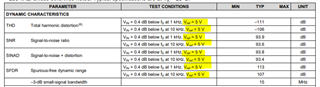If you have a related question, please click the "Ask a related question" button in the top right corner. The newly created question will be automatically linked to this question.

Hi sir,

There are REFIN and VREF in the data table. Are they the same?

If they are different, what are their differences.

If they are the same, why do they describe them separately?

In addition, if + VA = 5V,

What are the advantages and disadvantages of VREF = 4.096V and VREF = 5V?

Regards,

• Hello Frank,

REFIN and VREF can be treated as the same.  REFIN is the name of the pin on the physical device package, and VREF is the name of the voltage applied to the REFIN pin, where 2.25V ≤ VREF ≤ VA+0.1V.  Voltage applied to REFIN pin is equal to VREF.

The advantage of using VREF=5V is that it maximizes the dynamic range, or SNR of the ADC.  The internal noise of the ADC is fixed, and a larger voltage applied to the REFIN pin allows a larger input signal.  SNR=20*log(Vfs/Vnoise), where Vnoise is fixed and Vfs=VREF.  All of the Dynamic Characteristics for the ADS8339 are specified with VREF=5V for this reason.  If using a lower voltage for VREF, the SNR will be lower.The reason to use a lower voltage for VREF is to more closely match the input range of the signal that you want to measure.  A popular value for VREF is 4.096V, since this voltage is equal to 2^12 mV, 2^12=4096.

For a 16b converter with a full scale input range of Vfs=Vref=4.096, the size of the LSB in volts is:

LSB=Vref/2^N = 4.096/2^16 = 62.5μV.

Regards,
Keith Nicholas